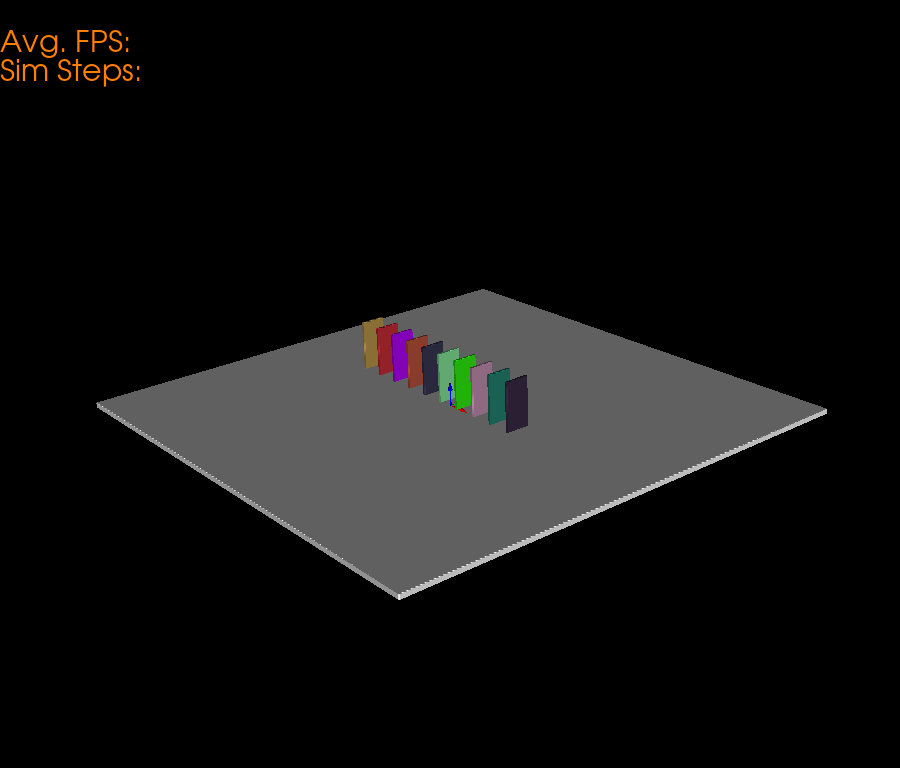# Domino Physics Simulation¶

This example simulation shows how to use pybullet to render physics simulations in fury. In this example we specifically render a series of Dominoes which are under Domino Effect.

First some imports.

```import numpy as np
from fury import window, actor, ui, utils
import itertools
import pybullet as p

# Next, we initialize a pybullet client to render the physics.
# We use `DIRECT` mode to initialize pybullet without a GUI.
client = p.connect(p.DIRECT)

# Apply gravity to the scene.
p.setGravity(0, 0, -10, physicsClientId=client)
```

Set the Number of Dominoes for Simulation.

```number_of_dominoes = 10

# Base Plane Parameters
base_size = np.array([number_of_dominoes*2, number_of_dominoes*2, 0.2])
base_color = np.array([1, 1, 1])
base_position = np.array([0, 0, -0.1])
base_orientation = np.array([0, 0, 0, 1])

# Render a BASE plane to support the Dominoes.
base_actor = actor.box(centers=np.array([[0, 0, 0]]),
directions=[0, 0, 0],
scales=base_size,
colors=base_color)

# half of the actual size.
base_coll = p.createCollisionShape(p.GEOM_BOX,
halfExtents=base_size / 2)

base = p.createMultiBody(
baseCollisionShapeIndex=base_coll,
basePosition=base_position,
baseOrientation=base_orientation)

p.changeDynamics(base, -1, lateralFriction=1, restitution=0.5)
```

We define some global parameters of the Dominoes so that its easier for us to tweak the simulation.

```domino_mass = 0.5
domino_size = np.array([0.1, 1, 2])

domino_centers = np.zeros((number_of_dominoes, 3))

# Keeping all the dominos Parallel
domino_directions = np.zeros((number_of_dominoes, 3))
domino_directions[:] = np.array([1.57, 0, 0])

domino_orns = np.zeros((number_of_dominoes, 4))

domino_sizes = np.zeros((number_of_dominoes, 3))
domino_sizes[:] = domino_size

domino_colors = np.random.rand(number_of_dominoes, 3)

domino_coll = p.createCollisionShape(p.GEOM_BOX,
halfExtents=domino_size / 2)

# We use this array to store the reference of domino objects in pybullet world.
dominos = np.zeros(number_of_dominoes, dtype=np.int8)

centers_list = np.zeros((number_of_dominoes, 3))

for i in range(number_of_dominoes):
center_pos = np.array([(i*0.99)-5.5, 0.4, 1])
domino_centers[i] = center_pos
domino_orns[i] = np.array([0, 0, 0, 1])
dominos[i] = p.createMultiBody(baseMass=domino_mass,
baseCollisionShapeIndex=domino_coll,
basePosition=center_pos,
baseOrientation=domino_orns[i])
p.changeDynamics(dominos[i], -1, lateralFriction=0.2, restitution=0.1)

domino_actor = actor.box(centers=domino_centers,
directions=domino_directions,
scales=domino_sizes,
colors=domino_colors)
```

Now, we define a scene and add actors to it.

```scene = window.Scene()

# Create show manager.
showm = window.ShowManager(scene,
size=(900, 768), reset_camera=False,
order_transparent=True)

showm.initialize()

# Counter iterator for tracking simulation steps.
counter = itertools.count()

# Variable for tracking applied force.
apply_force = True
```

Now, we define methods to sync objects between fury and Pybullet.

```# Get the position of base and set it.
base_pos, _ = p.getBasePositionAndOrientation(base)
base_actor.SetPosition(*base_pos)

# Calculate the vertices of the dominos.
vertices = utils.vertices_from_actor(domino_actor)
num_vertices = vertices.shape
num_objects = domino_centers.shape
sec = int(num_vertices / num_objects)
```

# Syncing Dominoes¶

Here, we perform three major steps to sync Dominoes accurately. * Get the position and orientation of the Dominoes from pybullet. * Calculate the Rotation Matrix.

• Get the difference in orientations (Quaternion).

• Generate the corresponding rotation matrix according to that difference.

• Reshape it in a 3x3 matrix.

• Perform calculations to get the required position and orientation.

• Update the position and orientation.

```def sync_domino(object_index, multibody):
pos, orn = p.getBasePositionAndOrientation(multibody)

rot_mat = np.reshape(
p.getMatrixFromQuaternion(
p.getDifferenceQuaternion(orn, domino_orns[object_index])),
(3, 3))

vertices[object_index * sec: object_index * sec + sec] = \
(vertices[object_index * sec: object_index * sec + sec] -
domino_centers[object_index]) @ rot_mat + pos

domino_centers[object_index] = pos
domino_orns[object_index] = orn
```

Here, we define a textblock to display the Avg. FPS and simulation steps.

```fpss = np.array([])
tb = ui.TextBlock2D(text="Avg. FPS: \nSim Steps: ", position=(0, 680),
font_size=30, color=(1, 0.5, 0))
```

Set the camera for better visualization.

```scene.set_camera(position=(10.46, -8.13, 6.18),
focal_point=(0.0, 0.0, 0.79),
view_up=(-0.27, 0.26, 0.90))
```

Timer callback is created which is responsible for calling the sync and simulation methods.

```# Create timer callback which will execute at each step of simulation.
def timer_callback(_obj, _event):
global apply_force, fpss
cnt = next(counter)
showm.render()

if cnt % 1 == 0:
fps = scene.frame_rate
fpss = np.append(fpss, fps)
tb.message = "Avg. FPS: " + str(np.round(np.mean(fpss), 0)) + \
"\nSim Steps: " + str(cnt)

# Get the position and orientation of the first domino.
domino1_pos, domino1_orn = p.getBasePositionAndOrientation(dominos)

# Apply force on the First Domino (domino) above the Center of Mass.
if apply_force:
# Apply the force.
p.applyExternalForce(dominos, -1,
forceObj=[100, 0, 0],
posObj=domino1_pos + np.array([0, 0, 1.7]),
flags=p.WORLD_FRAME)
apply_force = False

# Updating the position and orientation of individual dominos.
for idx, domino in enumerate(dominos):
sync_domino(idx, domino)
utils.update_actor(domino_actor)

# Simulate a step.
p.stepSimulation()

# Exit after 300 steps of simulation.
if cnt == 300:
showm.exit()

# Add the timer callback to showmanager.
# Increasing the duration value will slow down the simulation.

interactive = False

# start simulation
if interactive:
showm.start()

window.record(scene, out_path="viz_domino.png", size=(900, 768))
```Total running time of the script: ( 0 minutes 0.169 seconds)

Gallery generated by Sphinx-Gallery